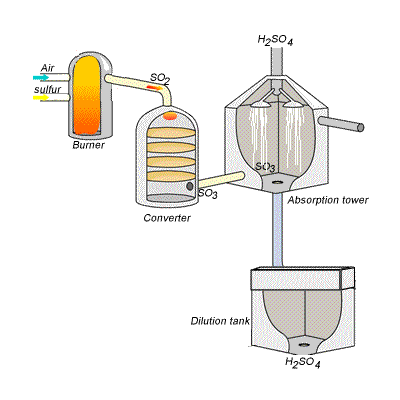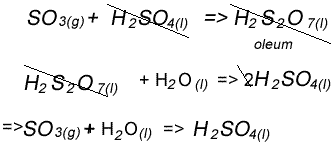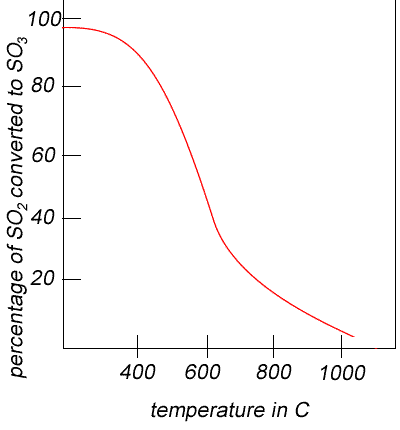Sulfuric acid - contact process Sulfuric acid can be produced by reacting sulfur trioxide directly with water according to the equation below. SO3(g) + H2O(l) =>H2SO4(aq) /\H = -130kJ mol- However, so much heat is released that the reaction chamber becomes full of sulfuric acid mist which is very difficult to collect. For this reason sulfuric acid is produced in several stages known as the contact process. Click on the blue writing to reveal the equations involved. Stage one - Liquid sulfur is sprayed in the burner where it reacts with dry air to produce sulfur dioxide (SO2). Water that may be present forms sulfuric acid in the converter and corrodes the wall. The air is dried using concentrated sulfuric acid. Stage two - The sulfur dioxide is oxidised to sulfur trioxide by oxygen using vanadium(V)oxide as a catalyst. Stage three - Concentrated sulfuric acid is used to dissolve sulfur trioxide where it forms oleum (H2S2O7) in an absorption tower. Stage four - Oleum is then mixed with water to obtain sulfuric acid.However the overall reaction, if you combine stage three and four, is shown on the right.In the converter sulfur dioxide is converted to sulfur trioxide. Sulfur dioxide is mixed with air and passed over several beds of loosely packed porous vanadium oxide catalyst. As shown above, the reaction is exothermic and heat quickly builds up in the converter reducing the equilibrium yield of this exothermic reaction. The graph on the right shows the equilibrium yield of the reaction at different temperatures. The reaction mixture in the converter is cooled as it passes from one catalyst bed to another and maintained at temperatures around 400oC to 500oC and 1 atm pressure.Using Le Chatelier's principle, the equilibrium yield of sulfur trioxide should increase when: - temperatures are low, since the reaction is exothermic; - pressure is high; - excess reactants are present. However the rate of the reaction is high when: - temperature is high, hence an obvious conflict exists with the equilibrium yield; - the pressure is high; - a catalyst is used. The conflict between increased yield and fast rate is resolved by the use of a catalyst. The use of a catalyst enables low temperatures to be used in the reaction vessel while maintaining a very high rate of reaction. The vanadium(v)oxide catalyst is made into a porous solid and layed out in several beds to increase the surface area exposed to the reactants. Keep in mind, a catalyst does not influence the equilibrium yield only the rate at which equilibrium is reached. The yield is further improved by using excess air in the reaction mixture and since the reaction occurs at atmospheric pressure the need for expensive, high pressure equipment is eliminated. In the converter sulfur trioxide is formed from sulfur dioxide and oxygen gas from the air. Give the expression for the equilibrium constant for this reaction. Solution Predict the reaction conditions that would favour maximum yield in the converter. Explain why Solution Predict the reaction conditions that would favour a high rate of reaction in the converter. Explain why Solution How are the conditions for maximum yield and rate in conflict with each other? A vanadium(V)oxide catalyst is used in the contact process. Why do we use a catalyst instead of simply raising the temperature to increase the rate of reaction? Why is sulfur trioxide not directly reacted with water to produce sulfuric acid? What is the main use for sulfuric acid in industry and why is it regarded as the most important industrial acid? Why must the air be dry before burning with molten sulfur? Calcium carbonate powder is placed in a dilute sulfuric acid solution. i) Give the balanced chemical equation for this reaction. ii) Give the ionic equation for this reaction. iii) Is sulfuric acid acting as an acid, oxidant or dehydrating agent? Explain. solution Calcium hydroxide solution is used to neutralise a dilute sulfuric acid solution. i) Give the balanced chemical equation for this reaction. ii) Give the ionic equation for this reaction. iii) Is sulfuric acid acting as an acid, oxidant or dehydrating agent? Explain. solution Click if you need help with ionic equations